• 代码： fig = pyp.figure(0) pyp.imshow(image, cmap=“gray”) ...fig.savefig(save_path, transparent=False, dpi=dpi, pad_inches=0) fig.clear() 其中fig.set_size_inches里面的x和y，乘以dpi就是最后保存图片像素
代码：
fig = pyp.figure(0) pyp.imshow(image, cmap=“gray”) pyp.figure(num=0) for j in range(len(coor)): pyp.scatter(coor[j]+shape,coor[j],s=10) pyp.axis(“off”) fig.set_size_inches(12,10) pyp.gca().xaxis.set_major_locator(pyp.NullLocator()) pyp.gca().yaxis.set_major_locator(pyp.NullLocator()) pyp.subplots_adjust(top=1, bottom=0, left=0, right=1, hspace=0, wspace=0) pyp.margins(0, 0) save_path=r"F:\fan/test.png" fig.savefig(save_path, transparent=False, dpi=dpi, pad_inches=0) fig.clear()
其中fig.set_size_inches里面的x和y，乘以dpi就是最后保存图片像素
展开全文图像识别
• import timeimport matplotlib.image as mpingdef getPngSize(pngPath ="aa.png"): print("获取图片尺寸大小："+ str(pngPath)) try: lena = mping.imread(pngPath) except: time.sleep...
import time
import matplotlib.image as mping
def getPngSize(pngPath ="aa.png"):
print("获取图片尺寸大小："+ str(pngPath))
try:
except:
time.sleep(0.5)
print("读图出错，重读")
getPngSize(pngPath)
size = tuple(lena.shape)
print(size)
return size,size

print(getPngSize())
展开全文• Talk is cheap, show me the code. ...import matplotlib.pyplot as plt import numpy as np x = np.arange(0, 2 * np.pi, 0.01) y = np.sin(x) plt.figure(figsize=(2,3), dpi=300) plt.plot(x,y) plt.show() 在...
Talk is cheap, show me the code. 代码如下：
import matplotlib.pyplot as plt
import numpy as np
x = np.arange(0, 2 * np.pi, 0.01)
y = np.sin(x)
plt.figure(figsize=(2,3), dpi=300)
plt.plot(x,y)
plt.show()

在anaconda prompt中输入后运行结果截图如下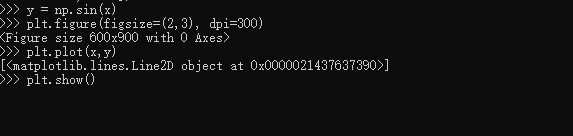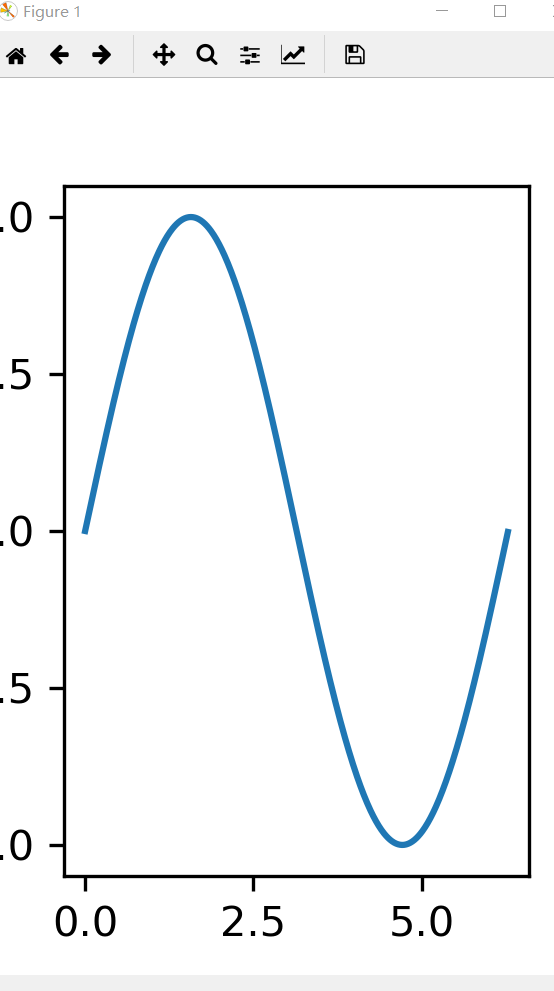figure图看起来很大，只是因为分辨率较高，像素点较多(600900)，将图片保存成png格式（矢量图）或eps格式（矢量图），然后复制到word中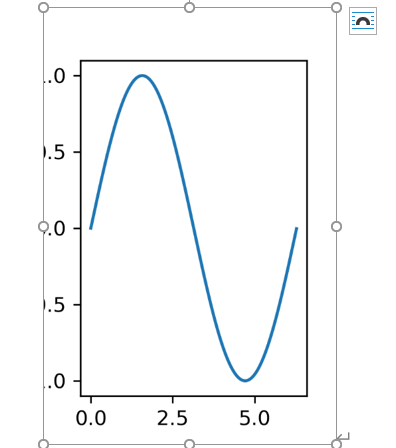图片的大小如下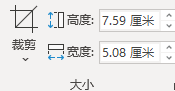这里解释一下，figsize=(2,3) 中2代表宽度，3代表高度，这里单位是inches(英寸)。注意：英寸(长度单位，等于2.54厘米，1英尺等于12英寸) 将英寸换算成厘米后，和word中显示的值基本一致（2.542=5.08；2.54*3=7.62，7.62和7.59差一点点，但误差在小数点后的第二位，可以接受）。于是在matplotlib中画图，并且控制图片尺寸就完成了。
下面是重点！！！！！ 下面是重点！！！！！ 下面是重点！！！！！
千万不要把spyder中画的图，直接复制到word中，spyder显示的图片会进行缩放，复制到word中尺寸是不对的。 在实际应用中，用Pycharm就可以轻松地控制图片的尺寸，而且不用像刚刚那样在命令行里一行一行地敲
展开全文• 图片与子片，调整子图周围的间距
matplotlib API入门plot(x, y, 'go--', linewidth=2, markersize=12)   # color marker linsstyle

**Markers**
=============    ===============================
character        description
=============    ===============================
'.'          point marker
','          pixel marker
'o'          circle marker
'v'          triangle_down marker
'^'          triangle_up marker
'<'          triangle_left marker
'>'          triangle_right marker
'1'          tri_down marker
'2'          tri_up marker
'3'          tri_left marker
'4'          tri_right marker
's'          square marker
'p'          pentagon marker
'*'          star marker
'h'          hexagon1 marker
'H'          hexagon2 marker
'+'          plus marker
'x'          x marker
'D'          diamond marker
'd'          thin_diamond marker
'|'          vline marker
'_'          hline marker
=============    ===============================

**Line Styles**

=============    ===============================
character        description
=============    ===============================
'-'          solid line style
'--'         dashed line style
'-.'         dash-dot line style
':'          dotted line style
=============    ===============================

**Colors**

The supported color abbreviations are the single letter codes

=============    ===============================         # 颜色也可以是16进制的颜色代码
character        color
=============    ===============================
'b'          blue
'g'          green
'r'          red
'c'          cyan
'm'          magenta
'y'          yellow
'k'          black
'w'          white
=============    ===============================

1.1 图片与子片
matplotlib所绘制的图位于图片（Figure)对象中，，可以使用 plt.figure生成一个新的图片：fig=plt.figure() 就会出现一个空的绘图窗口，我们可以使用一些命令来显示，比如 figsize 确保图片有一个确定的大小以及储存到硬盘时的长宽比。
figure参数含义num( int or str)图形的编号，默认是递增的，如果编号存在，就是对其的引用，如果是字符串，图形编号就是这个figsize(float,float)宽度，高度，单位英寸。dpi默认100，分辨率facecolor背景颜色edgecolor边框颜色frameon布尔值，默认True, 如果False,禁止绘制图形框。
不能使用空白的图片进行绘图，需要使用add_subplot 创建一个或多个子图（subplot） ax1=fig.add_subplolt(2,2,1)
意思是图片应该是 2X2 的（最多四个图形），并且我们选择了四个图形中的第一个（序号从1开始）。如果你接着创建了多个子图，你会看到：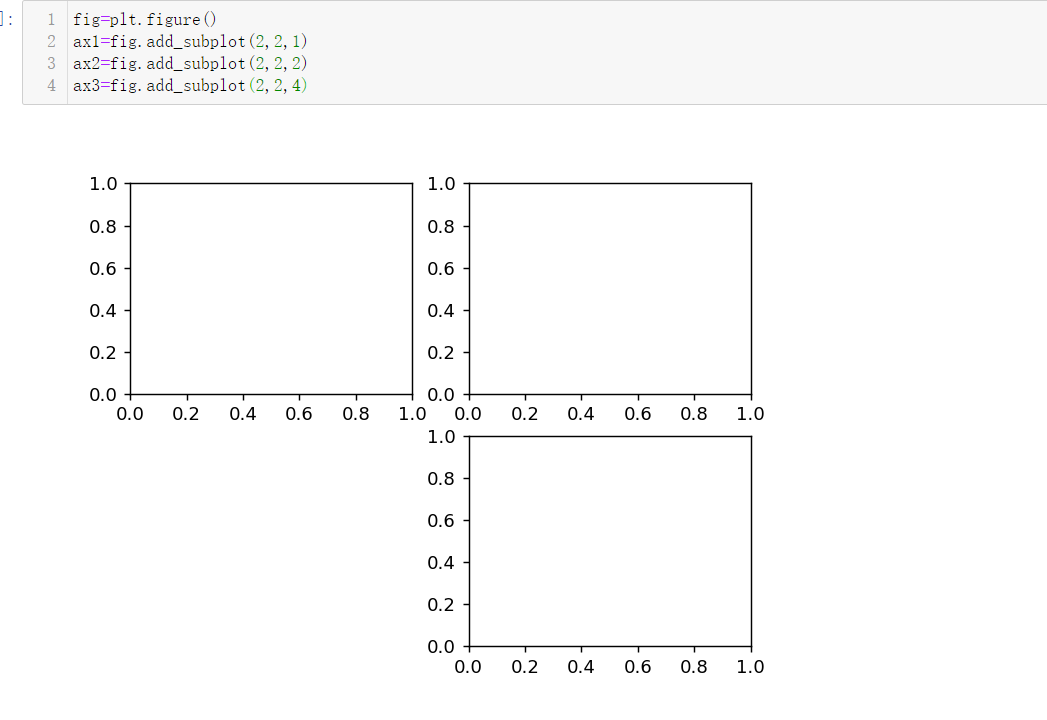（jupyter notebook 的每个单元格运行后，图标都会重置，对复杂的图标，必须将所有的绘图命令放到单个单元格中。）额，，把最后那个ax3=fig.add_subplot(2,2,4) 放到下一个格子运行，还是会放到这个图片里的，可以自己试试啊。。。。
输入 plt.plot() 命令会在最后一个图片和子图上进行绘制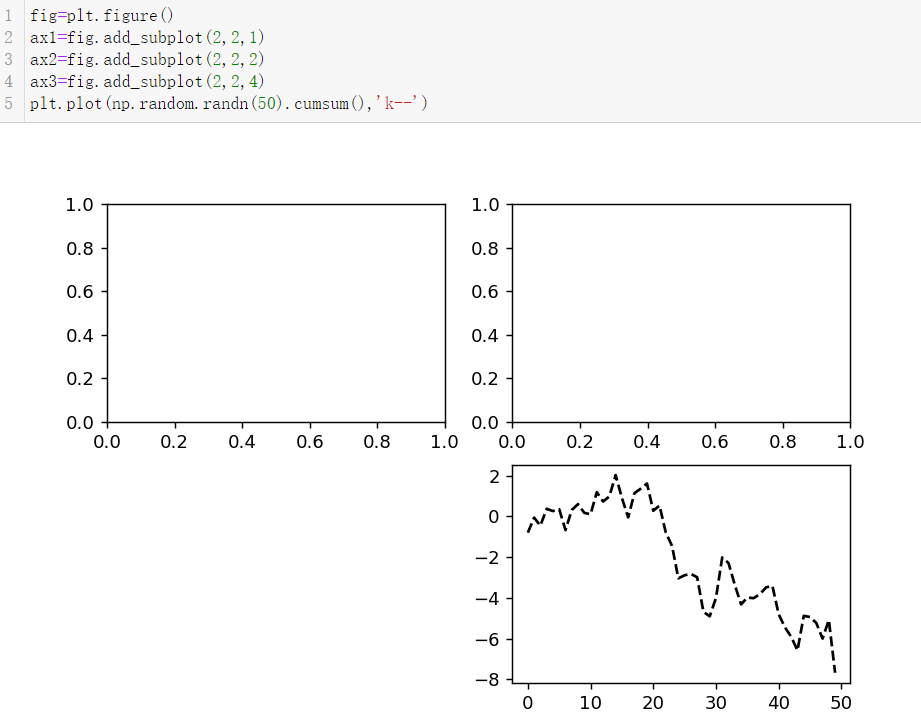’ k–’ 是用于绘制黑色虚线的 style 选项，fig.add_subplot 返回的对象是Axes Subplot 对象，使用这些对象你可以直接在其他空白的子图上调用对象的实例方法进行绘图。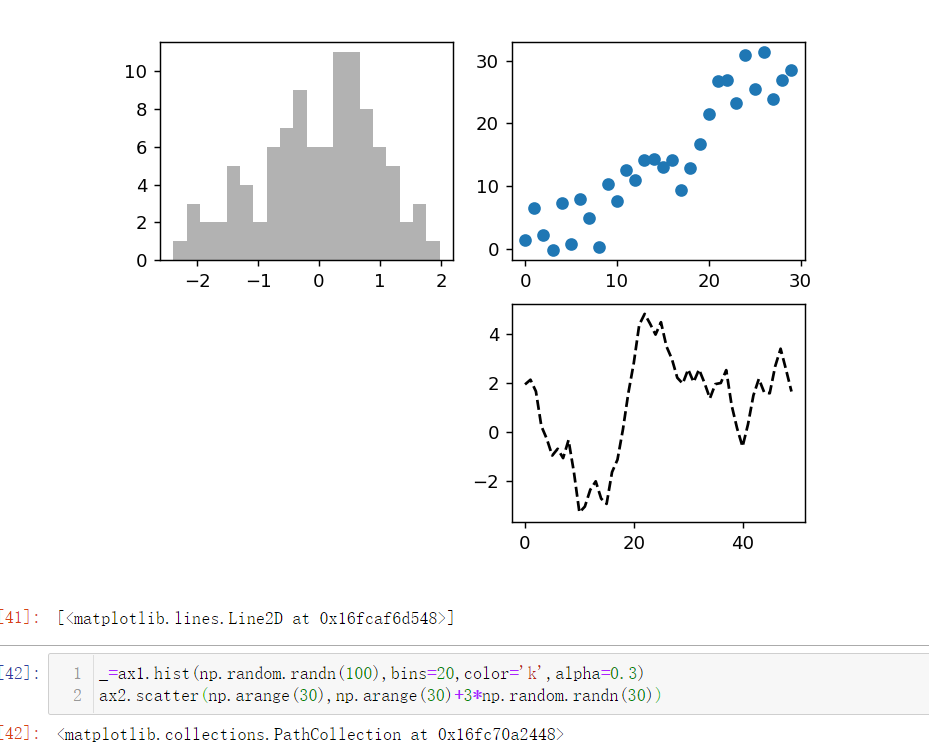就是柱状图和离散分布，，完整图形类型去看 matplotlib 的文档吧，，，脑补连接
plt.subplots 创建一个新的图片，然后返回包含了已生成子图对象的numpy数组，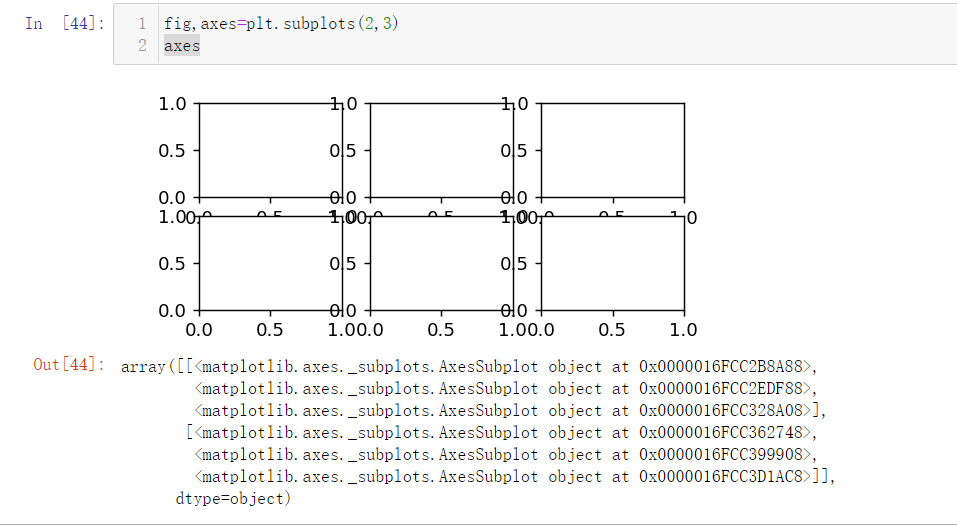这是很有用的，因为axes 可以像二维数组那样进行索引，axes[0,1] 也可以通过使用 sharex and sharey 来表明子图分别拥有相同的x y 轴。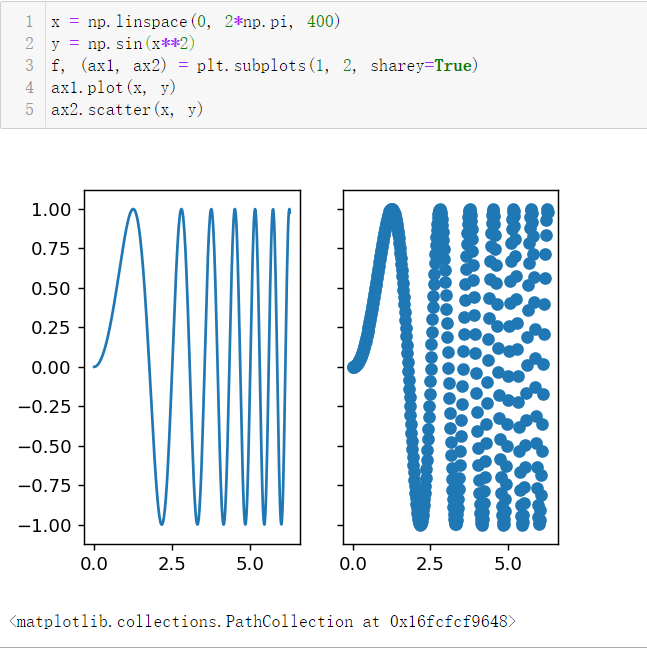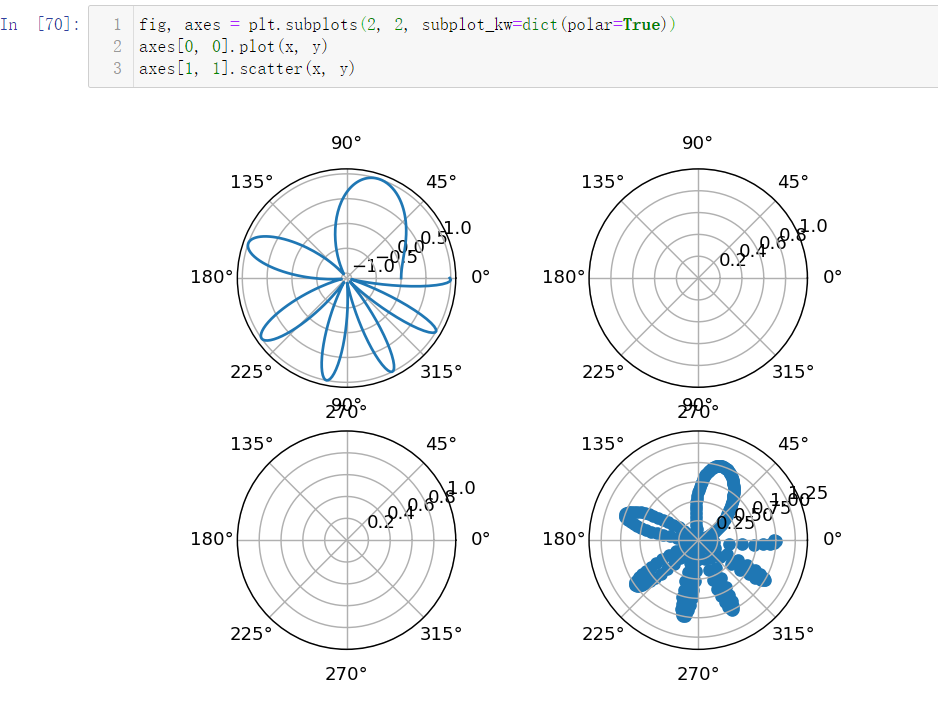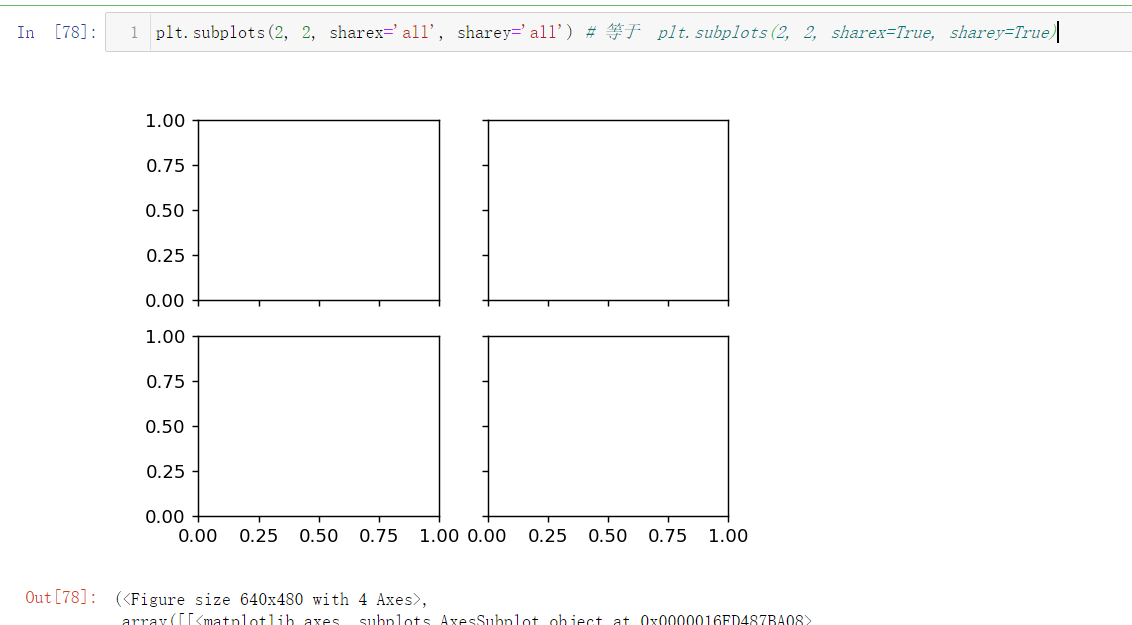1.1.1 调整子图周围的间距
默认情况下，会在子图的外部和子图之间留出一定间距，这个间距时根据图的高度和宽度调整的， subplots_adjust(left=None,bottom=None,right=None,top=None,wspace=None,hspace=None) 调整间距 wspace,hspace 控制的是图片的宽度和高度的百分比，以用作子图间的间距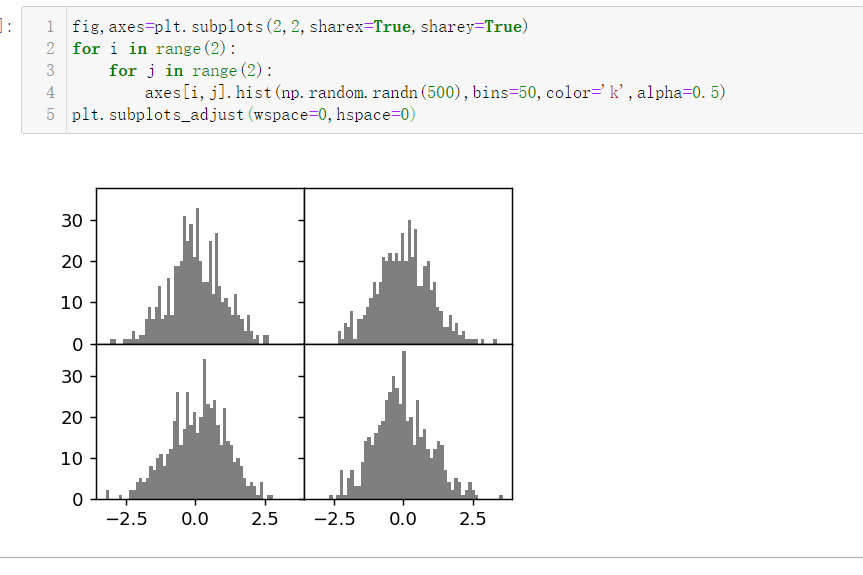展开全文数据分析
• 插入背景图片 matplotlib怎么修改背景颜色？ 1.通过matplotlib的相关参数修改 使用matplotlib画图时，可以通过rcParams['axes.facecolor']来修改背景颜色 import matplotlib.pyplot as plt # 修改背景颜色 plt....python
• 05-Matplotlib中图像的尺寸和分辨率 上一篇详细讨论了显示器的尺寸、分辨率，PPI，DPI的概念，在这个基础上，我们讨论图像的尺寸和分辨率就要容易得多了，如果你还没有阅读上一篇，而对这些概念又不是非常、非常地...
• 本文实例讲述了Python使用matplotlib 画矩形的三种方式。分享给大家供大家参考，具体如下： 假设矩形两点坐标如下，分别为：x1, y1, x2, y2 cat_dict['bbox'][i] = (min_row, min_col, max_row, max_col) 1. plt....
• matplotlib 使用 plt.savefig() 输出图片去除旁边的空白区域python
• plt.legend()#显示图例 plt.show()#使用该函数进行显示 （二）柱状图 柱状图的绘制需要用到bar函数 bar( x, y, width, facecolor, edgecolor, label ) 参数 解释 x x轴的序列个数 y y 条纹高度 width 条纹宽度 ...
• 有时我们在使用matplotlib作图时，图片不清晰或者图片大小不是我们想要的，例如下图 上图的相关代码如下： import matplotlib.pyplot as plt x = [10,20,30,40,50,60,70,80,90,1000] y1 = [7,17,27,37,43,49,57,65...python
• import matplotlib.pyplot as plt fig_width = 16/2.54 # 宽度16cm fig_height = 8/2.54 # 高度8cm dpi = 300 # 指定保存的dpi用于保存图片时候使用 fig, ax = plt.subplots( nrows=1, ncols=1, figsize=(fig_...python
• 参考自Matplotlib Python 画图教程 (莫烦Python)(13)_演讲•公开课_科技_bilibili_哔哩哔哩..."""输出栅格图片""" import numpy as np import matplotlib.pyplot...
• 前一篇文章讲述了数据预处理、Jieba分词和文本聚类知识，这篇文章主要介绍Matplotlib和Pandas扩展包绘图的基础用法，同时引入Echarts技术，该技术主要应用于网站可视化展示中。本文内容以实例为主，给读者最直观的...可视化分析 Python Pandas Echarts
• 文章目录matplotlib概述matplotlib基本功能matplotlib基本功能详解基本绘图绘图核心API线型、线宽和颜色设置坐标轴范围设置坐标刻度设置坐标轴图例特殊点备注图形对象（图形窗口）子图刻度定位器刻度网格线半对数...
• import matplotlib.pyplot as plt n = 102 #散点的个数 X = np.random.normal(0, 1, n) #生成散点的横坐标，这些数值服从均值为0，方差为1的正态分布 Y = np.random.normal(0, 1, n) T = np.arctan2(Y, X) #设定每...
• matplotlib与seaborn画图 matplotlib中pyplot画图 1.导入pyplot库 通过import matplotlib.pyplot as plt指令导入pyplot库并且命名为plt，之后就可以在程序中使用该库了。 2. 画一个sin函数的图像(折线图)...seaborn python
• ## matplotlib简介

千次阅读 2020-07-15 19:56:00
欢迎关注”生信修炼手册”!丰富的第三方模块赋予了python强大的能力，matplotlib作为python最流行的可视化模块之一，功能强大，用法简便。对于新手而言，其上手难度低，仅需...可视化 python 数据可视化 编程语言 数据分析
• # matplotlib是python中画图的一个包 ##在使用之前需要导包 import matplotlib as plt # 导入数据计算的包 import numpy as np ...#在同一个图片上绘制多个图片 plt.figure(figsize=,num=) # 控制图线条的颜色，
• ax.plot(x_date, y_date, 'r-', lw=5) #可以在这之前更新x_data和y_data的数据 plt.pause(0.1) #绘图暂停0.1s plt.pause(0) #防止图片自动关闭 2） 方法二 from matplotlib.animation import FuncAnimation fig = ...
• import matplotlib.pyplot as plt from numpy import * from mpl_toolkits.mplot3d import Axes3D #设置坐标轴 fig=plt.figure() #定义一个窗口 ax=Axes3D(fig) #在窗口添加一个3d坐标轴 #设置X,Y,Z的值 X=a...
• matplotlib基础 一、介绍 Matplotlib是python最著名的绘图库，它提供了一整套和matlab相似的命令和API，十分适合交互式地进行制图。而且也可以方便的将它作为绘图空间，嵌入GUI应用程序中。 Mtaplotlib库由各种...python 可视化
• 数据可视化已经被用于工作科研的方方面面，特别在数学建模，python是不二之选，Matplotlib 是 Python 最著名的绘图库，希望通过学习笔记总结，和大家一起学会绘图。python
• 一，绘制线性图。用于画抛物线，直线，折线等。...import matplotlib.pyplot as plt dx = np.arange(0,10,1) #array([0, 1, 2, 3, 4, 5, 6, 7, 8, 9]) dy = pow(dx,2) #array([ 0, 1, 4, 9,...等高线
• 应用Matplotlib的基本功能实现图形显示 应用Matplotlib实现多图显示 应用Matplotlib实现不同画图种类 1. Matplotlib之HelloWorld 1.1 什么是Matplotlib 是专门用于开发2D图表（包括3D图表） 以渐进、交互式方式...
• # height: 高度 # plt.axes([left_bottom_x, left_bottom_y, width, height]) plt . axes ( [ 0.1 , 0.1 , 0.3 , 0.4 ] ) plt . text ( 0.5 , 0.5 , '1' , ha = 'center' , va = 'center' , size =...
• 柱状图是一种以长方形的长度为变量的表达图形的统计报告图，由一系列高度不等的纵向条纹表示数据分布的情况，用来比较两个或以上的价值（不同时间或者不同条件），只有一个变量，通常利用于较小的数据集分析。...数据分析
• 首先如何在python中安装matplotlib库呢？ 在cmd中使用命令 pip install matplotlib 任务目标： 代码实例： import matplotlib.pyplot as plt #加载matplotlib库的pyplot模块并起别名叫plt import numpy as np #再...python...